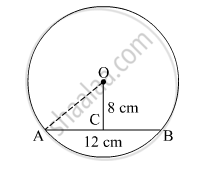Advertisement Remove all ads

# Distance of Chord Ab from the Centre of a Circle is 8 Cm. Length of the Chord Ab is 12 Cm. Find the Diameter of the Circle. - Geometry

Sum

Distance of chord AB from the centre of a circle is 8 cm. Length of the chord AB is 12 cm. Find the diameter of the circle.

Advertisement Remove all ads

#### SolutionLet the centre of the circle be O.
AB = 12 cm
OC = 12 cm
OC ⊥ AB
We know perpendicular drawn from the centre of the circle to the chord, bisects the chord.
So, AC = 6 cm
In   square OCA,

OC2 + AC2 = AO2

⇒ 82 + 62 = AO2

⇒ AO2 = 100

⇒ AO = 10

Thus, AO = 10 cm
Diameter = 2 × 10 cm = 20 cm.

Is there an error in this question or solution?
Advertisement Remove all ads

#### APPEARS IN

Balbharati Mathematics 2 Geometry 9th Standard Maharashtra State Board
Chapter 6 Circle
Practice Set 6.1 | Q 1 | Page 79
Advertisement Remove all ads
Advertisement Remove all ads
Share
Notifications

View all notifications

Forgot password?